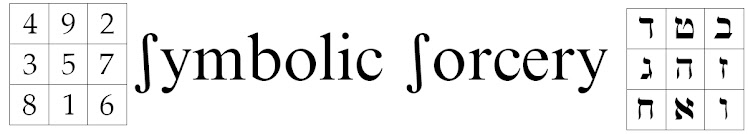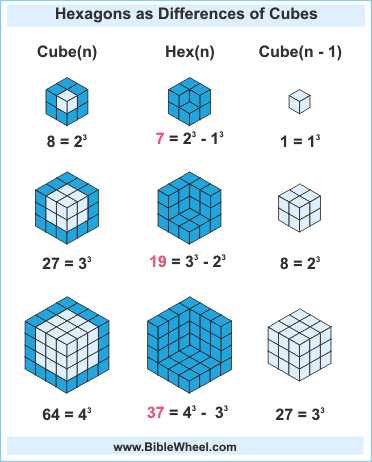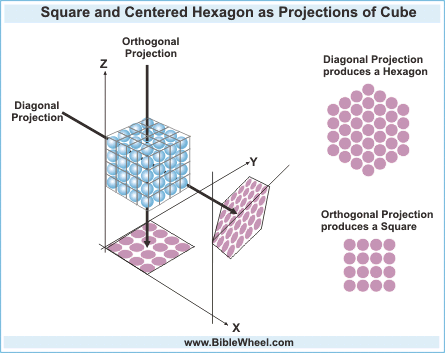## Tuesday, January 11, 2011

### Some Figurate Numbers and Gematria

A key area for studying the relationship between geometry and gematria is though the Figurate Numbers.  These archtypal integer sequences show patterns of dots in geometric configurations.  The number of dots in each configuration is an important key to gematria analysis.

A good example is the Centered Hexagonal Numbers:The number 37, the 4th Centered Hexagonal, has many interesting properties.

An interesting observation between 37 and multiples of 3:
111 = 37 * 3 and 1+1+1 = 3
222 = 37 * 6 and 2+2+2 = 6
333 = 37 * 9 and 3+3+3 = 9
444 = 37 * 12 and 4+4+4 = 12
555 = 37 * 15 and 5+5+5 = 15
666 = 37 * 18 and 6+6+6 = 18
777 = 37 * 21 and 7+7+7 = 21
888 = 37 * 24 and 8+8+8 = 24
999 = 37 * 27 and 9+9+9 = 27

Also, notice that 3*3*3 = 27 and 27 reduces to 9, which is 3*3.  Some other curios:

1+2+...+9 = 45.  45 reduces to 9.
1+2+...+45 = 2070 suggests again 27 and reduces to 9.
1+2+...+2070 = 2143485 which reduces to 27 (wow!)

There are 22 letters in the Hebrew alphabet, and if we also count the 5 finals we get 27.  There are 24 letters in the Greek alphabet plus 3 letters only used in arithmetic (digamma, quppa, sampi), also giving 27.

Consider the 27 small cubes shown below.  Only 26 of the small cubes can be seen from the outside, while there is One small cube in the center which is concealed.  26 = YHVH.An important aspect of the Centered Hexagonal Numbers is that they are differnces of Cube Numbers:Also, they can be seen as the diagonal projection of the Cube Numbers:By Greek gematria, 64 is the number of the word Alethia/Truth.

64 is the smallest figurate that is both a Square and a Cube.  As a Square, 64 = 8 * 8, so it relates to the Magic Square of Mercury, whose symbol gives 37.

37 is a Prime number, while 64 has only a single Prime factor, which is 2, the smallest Prime.

In decimal form, 64/37 = 1.729729729...

729 is the next smallest figurate after 64 that is both a Square and a Cube.  As a square, 729 = 27 * 27.  As a cube, 729 = 9 * 9 * 9.

Also by Greek gematria, 37 * 64 = 2368, which is the number of the letters in the name Jesus Christ.

There is also an occult correspondence between the Golden Ratio and the gematria of Jesus Christ.

By Greek gematria, Jesus = 888, while Christ = 1480.  Then Jesus Christ = 2368.

Now observe:
888 = 37 * 24
1480 = 37 * 40
2368 = 37 * 64

The proportion 24 : 40 : 64 is the same as 3 : 5 : 8 as can be seen by dividing by 8.  By Hebrew gematria, 358 = Messiah.

This shows the Golden Ratio in the name of the Messiah, which could be writen:

Jesus is to Christ as Christ is to Jesus Christ

One idea suggested by this is that: Humanity's aspirations to unite with God are like God's aspirations to create a Divinized Humanity.